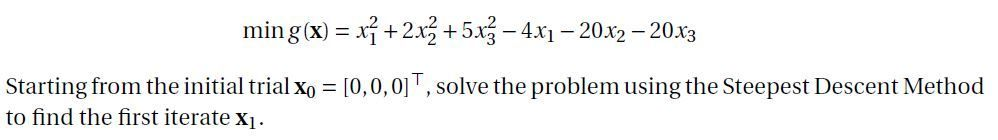## Fix code to calculate minimizer value in non linear optimization problem

hi,

I am trying to solve the following problemThe initial point is =(0 0 0)

My code is the following

```proc optmodel;
var x{1..3};
min f = x^2  + 2*x^2 + 5*x^2 - 4*x - 20*x - 20*x ;

/* starting point */
x= 0;
x= 0;
x=0;
solve;
print x x.dual;
quit;```

This code works fine

Now if P is the descent direction of f at Xo then

I need to find the minimizer Aplha for this problem

I mean to say that I am trying to solve this question:I have tried the following code to calculate Alpha

```proc optmodel;
num n = 3;
var x {1..n};
min f = x^2  + 2*x^2 + 5*x^2 - 4*x - 20*x - 20*x ;

/* starting point */
x= 0;
x= 0;
x=0;
solve;
print x x.dual;

num p {1..n};
for {j in 1..n} p[j] = -x[j].dual;
var alpha >= 0;
min g  = (x+alpha*p)^2 + 2*(x+alpha*p)^2 + 5*(x+alpha*p)^2-4*(x+alpha*p) -20*(x+alpha*p) -20*(x+alpha*p)
solve;
print alpha;
quit;```

But this code is giving me wrong value of alpha...its showing alpha=0

1 ACCEPTED SOLUTION

Accepted Solutions

## Re: Fix code to calculate minimizer value in non linear optimization problem

You still need to correct the first error by adding a FIX statement.

4 REPLIES 4

## Re: Fix code to calculate minimizer value in non linear optimization problem

There are three errors:

1. You need to FIX x for the first solve, as in the previous examples.

2. You need a semicolon at the end of the declaration of g.

3. Some of the appearances of p in g should instead be p or p.

The corrected statement is:

``````min g = (x+alpha*p)^2 + 2*(x+alpha*p)^2 + 5*(x+alpha*p)^2-4*(x+alpha*p) -20*(x+alpha*p) -20*(x+alpha*p);
``````

## Re: Fix code to calculate minimizer value in non linear optimization problem

Thanks for the quick response.

I fixed the code now like this:

```proc optmodel;
num n = 3;
var x {1..n};
min f = x^2  + 2*x^2 + 5*x^2 - 4*x - 20*x - 20*x ;

/* starting point */
x= 0;
x= 0;
x=0;
solve;
print x x.dual;

num p {1..n};
for {j in 1..n} p[j] = -x[j].dual;
var alpha >= 0;
min g = (x+alpha*p)^2 + 2*(x+alpha*p)^2 + 5*(x+alpha*p)^2-4*(x+alpha*p) -20*(x+alpha*p) -20*(x+alpha*p);

solve;
print alpha;
quit;```

but when I run it. I get the value of alpha=0. Is it ok or need some adjustment in the code please?

thanks

## Re: Fix code to calculate minimizer value in non linear optimization problem

You still need to correct the first error by adding a FIX statement.

## Re: Fix code to calculate minimizer value in non linear optimization problem

thank you so much..i fixed it like this

``````proc optmodel;
num n = 3;
var x {1..n};
min f = x^2  + 2*x^2 + 5*x^2 - 4*x - 20*x - 20*x ;

/* starting point */
fix x = 0;
fix x = 0;
fix x = 0;

solve;
print x x.dual;

num p {1..n};
for {j in 1..n} p[j] = -x[j].dual;
var alpha >= 0;
min g = (x+alpha*p)^2 + 2*(x+alpha*p)^2 + 5*(x+alpha*p)^2-4*(x+alpha*p) -20*(x+alpha*p) -20*(x+alpha*p);

solve;
print alpha;
quit;``````
Discussion stats
• 4 replies
• 444 views
• 0 likes
• 2 in conversation